V.6 No 1 23 Some improvements to the definition of entropy of macrosystemTo calculate, let us take some spherical volume of gas – e.g., molecular hydrogen, satisfying the Shklovsky condition (8). Premise the mass of this gas Mcloud = 10 M= 1,991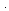1034 g  and its initial temperature T = 4o K. Then the radius of this sphere is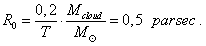(17)
 Premising that before compression the gas was evenly distributed in the volume, we can determine the initial gas pressure; we will need this value in calculation below. Noting the gas in interstellar clouds is very rarefied, we may think the gas ideal and determine its pressure with the laws of gas dynamics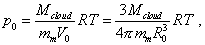(18)
 where R = 8,31696 j/ dgmole is the gas constant and mm = 21,0079 g/ mole is the molar mass of molecular hydrogen. Substituting the known values to (18), yield p0 = 2,1510-14 N/ m2 = 2,8610-12  mm of mercury. The yielded value of initial pressure corroborates the validity of initial premise to think the gas ideal.   Now suppose that the gas in the volume is not affected externally, it is quasi-statically compressed to the centre exceptionally under affection of its own gravity field. As the gas is isolated from external affection (as stipulated) and during the compression no one parameter of the gas continuum is constant, we can premise the adiabatic pattern of process. With it, at least at the stage of primary compression, the condition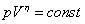(19)
 will be true, where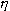is the Poisson constant. For a two-atomic gas like molecular hydrogen= 1,4. It will be desirable for further calculation to transform (19) to the relationship between the pressure and density of substance. Select some infinitesimal volume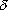v of compressed gas. Noting (19), the equality [19, p. 21] will be true for it: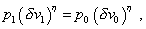(20)
 where p0 is the initial pressure in the selected volumev0 . With it, as the mass of gas in the volume did not change, the equality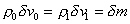(21)
 is true, where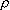0 is the initial gas density in the volumev0 andm is the mass if this gas volume. Joining (20) and (21), yield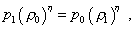(22)
 which is the sought relation. In finding this relation, we premised that all selected small volumes of the cloud are transformed during the substance redistribution. And we have to account, such transformations have to lead to the related shifts of these volumes along the radius. To account this factor, take some small selected volume with the height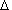r0i located at the distance r0i from the centre to which the cloud’s substance is shrunk, as in Fig. 4, and consider its shift to the radius  r1i with the transformation taking place at this time, to the heightr1i , noting the central symmetry of the problem.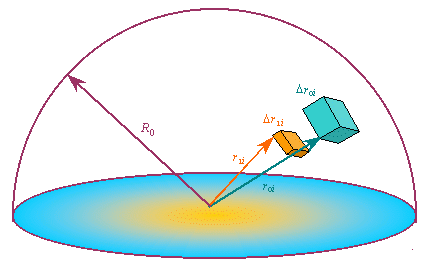Fig. 4. The calculation scheme of the selected volume in the cloud substance redistribution
 As the problem is centrally symmetrical and the selected volume is a part of some thin shell with the heightr0i located at the distance r0i from centre, we may broaden the analysis for this shell. We can write for it: if its height was initiallyr0i and after the substance redistributionr1i , and the shell has shifted to r1i , then in accordance with the adiabatic pattern of process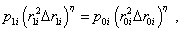(23)
 where p0i and p1i are the pressures in the volume before and after the cloud substance redistribution. Naturally, all elementary volumes of which the shell consists will transform after the same law; so we may further use (23) to study the elementary volume.   Then notice, r1i in (23) accounts that simultaneously with the selected volume transformation, there occurs also the transformation of all volumes along the cloud radius, as in absence of this accompanying transformation, the selected volume did not shift along the radius but only changed its height. But if after the redistribution the height of volumes located lower than that ith becamer1j , we can write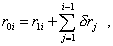(24)
 where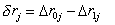(25)
 and is taken positive in the compression of selected volume.

Contents: / 18 / 19 / 20 / 21 / 22 / 23 / 24 / 25 / 26 / 27 /